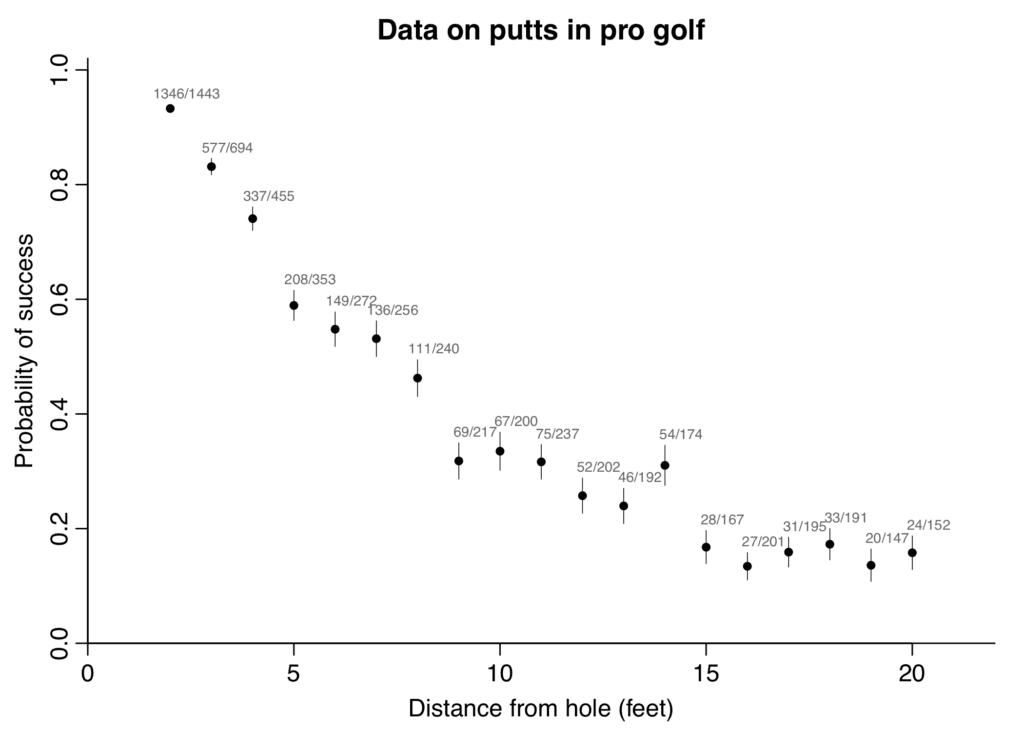# New golf putting data! And a new golf putting model!

Part 1

Here’s the golf putting data we were using, typed in from Don Berry’s 1996 textbook. The columns are distance in feet from the hole, number of tries, and number of successes:

```x n y
2 1443 1346
3 694 577
4 455 337
5 353 208
6 272 149
7 256 136
8 240 111
9 217 69
10 200 67
11 237 75
12 202 52
13 192 46
14 174 54
15 167 28
16 201 27
17 195 31
18 191 33
19 147 20
20 152 24
```

Graphed here:Here’s the idealized picture of the golf putt, where the only uncertainty is the angle of the shot:Which we assume is normally distributed:And here’s the model expressed in Stan:

```data {
int J;
int n[J];
vector[J] x;
int y[J];
real r;
real R;
}
parameters {
real sigma;
}
model {
vector[J] p;
for (j in 1:J){
p[j] = 2*Phi(asin((R-r)/x[j]) / sigma) - 1;
}
y ~ binomial(n, p);
}
generated quantities {
real sigma_degrees;
sigma_degrees = (180/pi())*sigma;
}
```

Fit to the above data, the estimate of sigma_degrees is 1.5. And here’s the fit:Part 2

The other day, Mark Broadie came to my office and shared a larger dataset, from 2016-2018. I’m assuming the distances are continuous numbers because the putts have exact distance measurements and have been divided into bins by distance, with the numbers below representing the average distance in each bin.

```x n y
0.28 45198 45183
0.97 183020 182899
1.93 169503 168594
2.92 113094 108953
3.93 73855 64740
4.94 53659 41106
5.94 42991 28205
6.95 37050 21334
7.95 33275 16615
8.95 30836 13503
9.95 28637 11060
10.95 26239 9032
11.95 24636 7687
12.95 22876 6432
14.43 41267 9813
16.43 35712 7196
18.44 31573 5290
20.44 28280 4086
21.95 13238 1642
24.39 46570 4767
28.40 38422 2980
32.39 31641 1996
36.39 25604 1327
40.37 20366 834
44.38 15977 559
48.37 11770 311
52.36 8708 231
57.25 8878 204
63.23 5492 103
69.18 3087 35
75.19 1742 24
```

Comparing the two datasets in the range 0-20 feet, the success rate is similar for longer putts but is much higher than before for the short putts. This could be a measurement issue, if the distances to the hole are only approximate for the old data.

Beyond 20 feet, the empirical success rates are lower than would be predicted by the old model. This makes sense: for longer putts, the angle isn’t the only thing you need to control; you also need to get the distance right too.

So Broadie fit a new model in Stan. See here and here for further details.